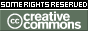# [#Script #Coding] Python TensorFlow for Machine Learning – Neural Network Text Classification Tutorial

### Python TensorFlow for Machine Learning – Neural Network Text Classification Tutorial

By freeCodeCamp.org
Published: Jun 15, 2022This course will give you an introduction to machine learning concepts and neural network implementation using Python and TensorFlow. Kylie Ying explains basic concepts, such as classification, regression, training/validation/test datasets, loss functions, neural networks, and model training. She then demonstrates how to implement a feedforward neural network to predict whether someone has diabetes, as well as two different neural net architectures to classify wine reviews.

Course created by Kylie Ying.
Instagram: https://instagram.com/kylieyying/

This course was made possible by a grant from Google’s TensorFlow team.

Course Contents

• (0:00:00) Introduction
• (0:00:34) Colab intro (importing wine dataset)
• (0:07:48) What is machine learning?
• (0:14:00) Features (inputs)
• (0:20:22) Outputs (predictions)
• (0:25:05) Anatomy of a dataset
• (0:30:22) Assessing performance
• (0:35:01) Neural nets
• (0:48:50) Tensorflow
• (0:50:45) Colab (feedforward network using diabetes dataset)
• (1:21:15) Recurrent neural networks
• (1:26:20) Colab (text classification networks using wine dataset)